``````
The Internal Structure of Crystals   (Part Sixteen)
```

```
back to homepage

The External Shapes of periodic 2-D arrays of repeated two-dimensional motifs

We continue our investigation concerning the faces, Forms and combinations of Forms in two-dimensional crystals.
In the previous Part we embarked onto the investigation of the 2-D Hexagonal Crystal System, and began with considering the Class 3 and its associated Plane Group P3.
Here we will continue that investigation.

Hexagonal Net. (continued)
At the end of the previous Part we depicted a crystal of the Class 3 representing one of the possible triangular Forms (as derived in Figure 3 in Part 15) of that Class. The crystal, as depicted, consisted of four hexagonal building blocks. Indeed it turned out that when we choose a (possible) building block from the hexagonal net in order to construct possible faces and show the possible atomic aspects presented to the environment by these faces, we must choose a building block that possesses the point symmetry of the present Class, which here means that the building block must display 3-fold rotational symmetry.
Initially we had chosen a rhombic building block, which is indeed neatly repeated throughout the structure but which does not have a 3-fold rotational point symmetry. That's why we could not, just like that, construct crystal faces by stacking such building blocks. But by using hexagonal building blocks we succeeded. We constructed the above described crystals, using four such building blocks. Of course this is a very small crystal indeed, but we can let it grow indefinitely by using ever more such hexagonal building blocks. See Figures 1 and 2.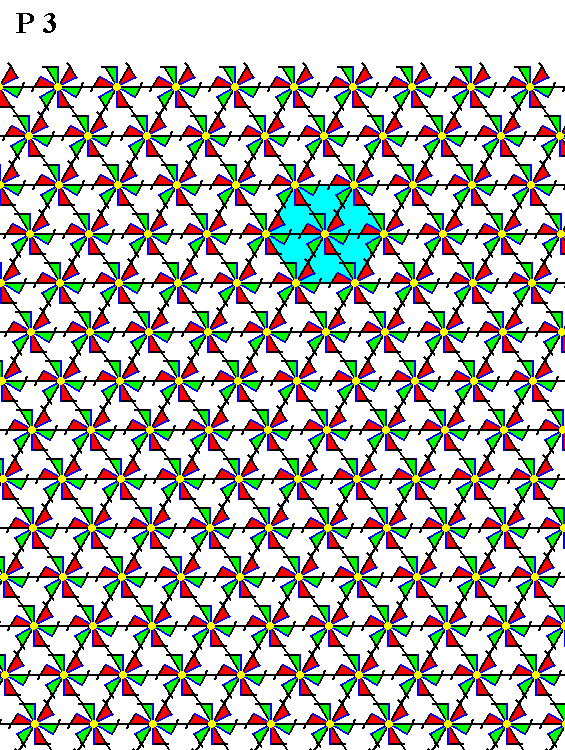Figure 1.  A periodic pattern of motifs representing Plane Group P3 (as given earlier, but now further extended). The net is drawn such that we can pick out hexagonal building blocks, for the construction of crystals and their faces. One such building block is indicated.

```
```
We will now again depict a somewhat larger representative of the triangular crystal of Figure 25 in the previous Part.Figure 2.  Indication of a 2-D crystal, as specified above, consisting of ten hexagonal building blocks ( See also next Figure ).

```
```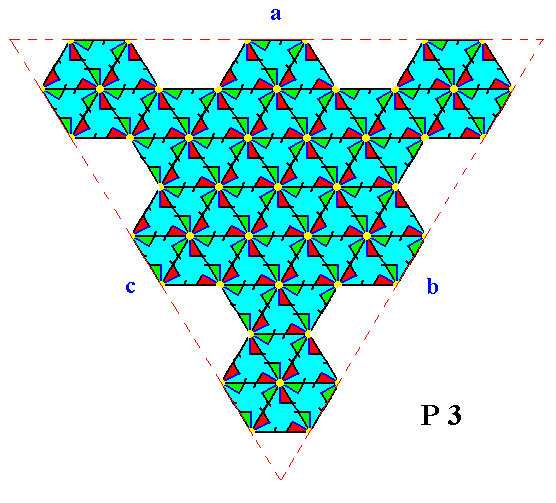Figure 3.   A two-dimensional crystal of the Class 3 of the 2-D Hexagonal Crystal System. The crystal represents one of the possible Forms of the Class and consists of ten hexagonal building blocks. The faces are indicated by a, b and c.

```
```
So because it has turned out that, in order to construct faces, Forms and crystals, we must use building blocks that have the full symmetry of the relevant Crystal Class, we will again set up the (same) hexagonal net, but in such a way that those appropriate building blocks (in our case hexagons) are clearly indicated. See the next Figures.Figure 4.  Construction of a hexagonal net that displays hexagonal building blocks, from the (equivalent) hexagonal net as depicted and used earlier.
(1). Hexagonal net, as used earlier. It depicts a repetition of rhombuses. The point symmetry of such a rhombus is not the same as that of the present Class (which is
3).
(2). Insertion of diagonal lines, running NW--SE (red) in the net of (1).
(3). The net as resulting from this insertion.
(4). This net supports
hexagonal building blocks. Some of these blocks are indicated.
(5). This image clearly shows that those building blocks can tile the plane completely, showing them to be genuine building blocks for crystals of the 2-D Hexagonal System.
(6). Removal of net elements (lines) in order to emphasize the hexagonal building blocks.

```
```
When we multiply the image of (6) of the above Figure, we obtain an extended hexagonal net, built up by the regular stacking of (regular) hexagons. See the next Figure.Figure 5.   A hexagonal net based on a regular hexagon as building block.

```
```
The hexagonal net, as given in Figure 5, will now be used to (again) establish possible crystal faces, Forms and combination of Forms, compatible with the present Crystal Class (3). Recall that possible faces must be such that they can be constructed by regularly stacking the building blocks, in our case the hexagonal building blocks. The edges of the stacking then represent possible crystal faces.
The position of the system of crystallographic axes remains the same as before, i.e. two of them coincide with the edges of a unit mesh. This unit mesh has still the shape of a rhombus (while the shape of the building block is a regular hexagon and as such consistent with the point symmetry of the present Class). See Figure 6.Figure 6.  Position and orientation of the system of crystallographic axes (red).
(1). The hexagonal net, as drawn earlier, with the axial system indicated. Two axes coincide with edges of the unit mesh (blue).
(2). The same net, but now diagonal lines are added, in order to construct an equivalent net with hexagons as building block. The unit mesh remains the same, it still has the shape of a rhombus (blue).
(3). A hexagonal building block (green) is indicated. The position of the axial system is unchanged.
(4). By removing part of the existing lines a hexagonal net is constructed emphasizing the hexagonal building blocks. The axial system has not changed position. Its center coincides with the center of a hexagonal building block. This net, and its axial system as here indicated, will now be used to construct possible crystal faces and Forms (a larger meshed version of it will be used to show the possible atomic aspects presented to the environment by those faces.

```
```
An introduced face parallel to one of the (equivalent) crystallographic axes is multiplied by 3 in virtue of the presence of the 3-fold rotation axis (as the only symmetry element of our Class (i.e. the Crystal Class 3). The resulting Form is an equilateral triangle (regular trigon) and its point symmetry complies with the symmetry of the Class. See Figure 7.Figure 7.   An initial face, parallel to one of the crystallographic axes implies two more (equivalent) faces, together making up a closed Form that has the shape of an equilateral triangle, and can as such represent a crystal of the present Class (3).
The 3-fold rotation axis (as the only symmetry element of our Class) is situated in the center of the system of crystallographic axes.

```
```Figure 8.   A crystal, based on the Form derived above, is indicated.
The triangular pattern of hexagons complies with a central 3-fold rotation axis, and so is consistent with the symmetry of our Class. But also mirror lines are clearly present in that pattern, because at this stage we have not yet furnished the net with compatible motifs (only then should the absence of mirror lines be appearant).
The yellow meshes serve to emphasize the 3-fold rotational symmetry of the triangular pattern. See the next Figure for the final result of the construction of the crystal.

```
```Figure 9.   A two-dimensional crystal of the Class 3 of the 2-D Hexagonal Crystal system, (the crystal) as specified above.
Because the building blocks are still empty, the crystal, as depicted, has the point symmetry of the most symmetric trigonal crystals. The latter have a point symmetry
3m, and indeed we see this point symmetry to be present : The crystal is mirror symmetric with respect to three mirror lines perpendicular to the crystallographic axes. But as soon as we furnish the building blocks with motifs compatible with the Class 3 (and consequently obtain a pattern representing its only Plane Group), the absence of the mirror lines will become appearant.

```
```
Introduction of a face that is perpendicular to one of the crystallographic axes will lead to a multiplication of that face by 3, in virtue of the action of the 3-fold rotation axis, resulting in a closed Form having the shape of an equilateral triangle (having a different orientation than the one derived above). This triangle can represent a 2-D crystal of the present Class.
See the next Figures.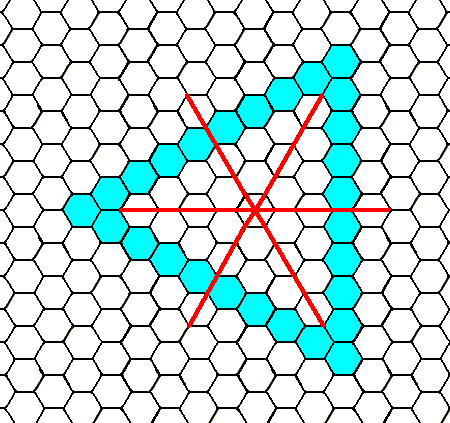Figure 10.  An initial face, perpendicular to one of the (equivalent) crystallographic axes implies two more faces, resulting in a closed Form having the shape of an equilateral triangle. The system of crystallographic axes (red) is indicated. This triangle can represent a 2-D crystal of the present Class (See next Figure).

```
```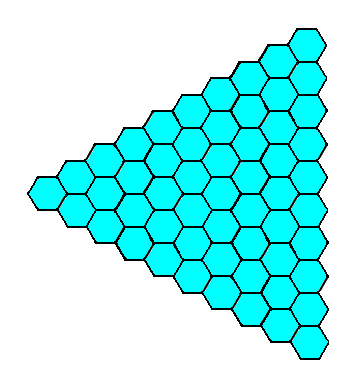Figure 11.   A two-dimensional crystal of the Class 3 of the 2-D Hexagonal Crystal System, (the crystal) as specified above.

```
```
Besides the faces we have seen so far, there are still other faces posible. See the next Figures.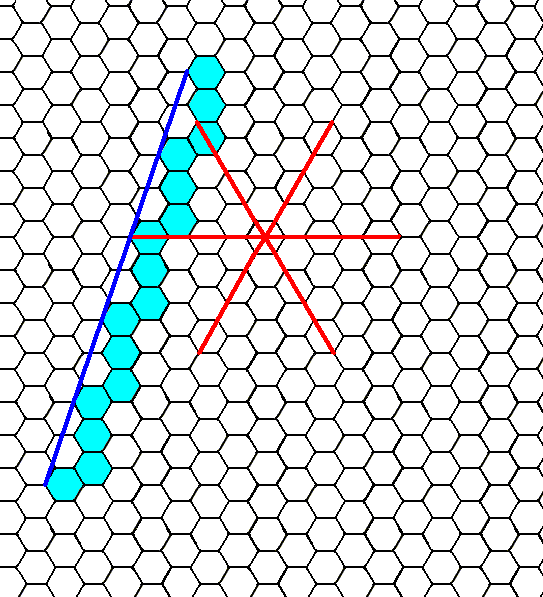Figure 11a.   A possible face of a crystal of the 2-D Hexagonal Crystal System.
This face is neither parallel nor perpendicular to any of the crystallographic axes. The heavy solid blue line helps to visualize this face.

```
```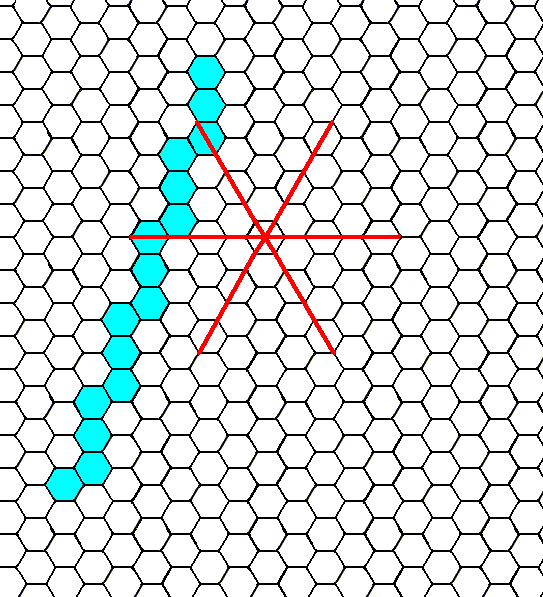Figure 11b.  The same as Figure 11a, but now with the auxiliary line omitted.

```
```
Introducing the above face in the context of Class 3 leads to the generation of two more faces in virtue of the 3-fold rotation axis, resulting in a closed Form having the shape of an equilateral triangle. See the next Figure.Figure 11c.  When the face, specified above, is subjected to the symmetry elements of the present Class -- which here means subjected to the action of the 3-fold rotation axis -- it is multiplied by 3, resulting in a closed Form having the shape of an equilateral triangle that can represent a crystal of this Class (See next Figure). This triangle has a different orientation -- with respect to the axial system -- from that of the previous derived triangles.

```
```Figure 11d.   A two-dimensional crystal of the Class 3, derived from the face specified above.

```
```
The above derived Forms, not only each by themselves can represent crystals of our Class, they also can enter in combinations. The next Figure depicts a crystal representing such a combination.Figure 12.   A two-dimensional crystal of the Class 3 of the 2-D Hexagonal Crystal System. It is a combination of two of the above derived Forms : From the crystal (Form) of Figure 9 the corners are cut off by the crystal (Form) of Figure 11. And here, already before having inserted compatible motifs, we see that the true symmetry of the present Class is revealed :  while the above equilateral triangles suggest mirror lines to be present, here we see that they actually are not present. The only symmetry element that is present is a 3-fold rotation axis, consistent with the Class 3. Indeed, real (i.e. 3-dimensional) crystals sometimes suggest a certain high symmetry, but when crystals are found consisting of combinations of Forms, the true (lower) symmetry is revealed.

```
```
While having derived faces, Forms and combinations, we will now look to the possible atomic aspects presented to the growing environment by those faces. This means that we are going to furnish the empty building blocks with motifs that are compatible -- with respect to their symmetry -- with the present Class (3). Furnishing the net with motifs of one or another symmetry type (compatible with the point symmetry of the Class) yields possible Plane Groups. In our case, i.e. for the Crystal Class 3, there is only one unique Plane Group, namely P3.
The atomic aspects as presented to the environment by the faces of the Form (crystal) of Figure 9 were already given earlier See Figure 3.

The atomic aspects presented to the environment by the faces of the Form (crystal) of Figure 11 are considered in the next Figures.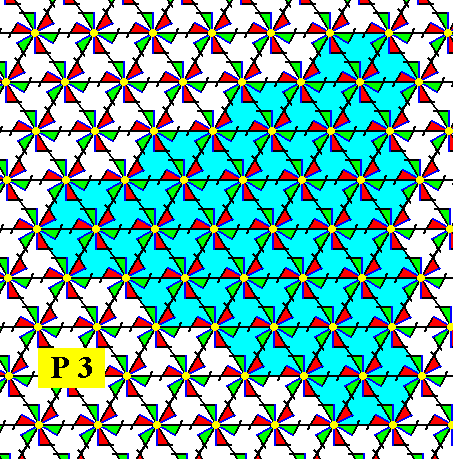Figure 13.   A triangular pattern representing Plane Group P3, bounded by the faces as derived in Figure 10. From this we will construct the atomic aspects presented to the environment by those faces. See the next Figure.

```
```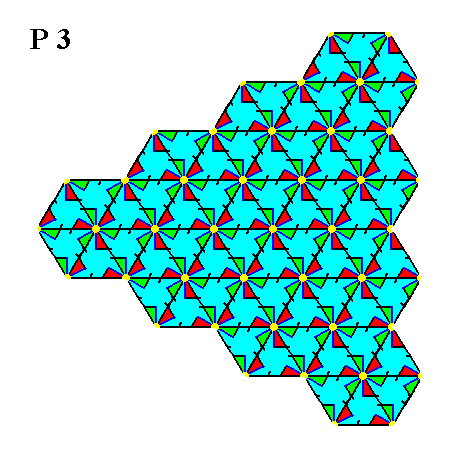Figure 14.   Atomic aspects presented to the environment by the faces of a 2-D crystal (as specified above). The incomplete motifs at the faces express unsatisfied or distorted chemical valences. The crystal belongs to Class 3 of the 2-D Hexagonal Crystal System. Its internal structure represents Plane Group P3.

```
```
In Figure 14, as well as in Figure 3, we can clearly see the presence of a 3-fold rotation axis (mapping the triangular pattern onto itself after every 1200 rotation of that triangular pattern). We can also see the absence of mirror lines, so indeed this structure is consistent with the Class's point symmetry (3).
```
```
To show that the triangular pattern of Figure 14 indeed possesses a 3-fold rotation axis expressing its point symmetry, I have rotated the image of Figure 14 clockwise by 1200. As can be observed, the rotated image is identical to the original (non-rotated) image.
See Figure 15.Figure 15.  The image of Figure 14, after rotation by 1200.
Both images are identical, showing the presence of a 3-fold rotation axis.
The same will apply with repect to the rotation of the image of Figure 3.

```
```
The next Figures consider the atomic aspect of the face derived in Figures 11a and 11b, and as seen in the Form depicted in the Figures 11c  and 11d.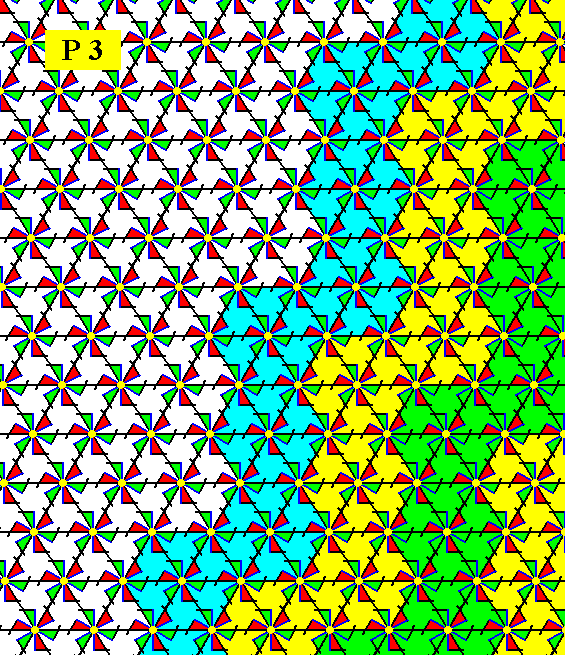Figure 16.   Indication -- but not yet a full construction -- of the atomic aspect of the face specified above (and depicted in Figure 11a).

```
```Figure 17.   Atomic aspect presented to the environment by the face specified above.

```
```Figure 18.   The same as Figure 17, but now with some coloration removed in order to highlight the motifs. The face is indicated by a.
The Class is
3, and the Plane Group is P3.

```
```
This concludes our exposition of the faces, Forms and atomic aspects associated with the Class 3 of the 2-D Hexagonal Crystal System.
In the next Part we will consider the second Class of this System, namely the Class 3m.
```

```
```